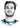# How are generators transpiled to ES5

Category:

I was curious how ES7’s `async/await` works under the hood, but then I quickly realized this goes deeper than I had initially thought. It’s a good idea to take a look at ES6’s `generators` first in this post.

## What are JavaScript `generators`

A generator is a type of functions in JavaScript that can return at specified points, stopping their execution, and which can later be continued again. Legend says they can be used to write asynchronous code in a more straight-forward manner. At least once you got used to their, admittedly, weird syntax which looks like this:

``````function *add(x) {
let y = yield(x*5)
return x + y
}

// generators are executed through iterators
let iterator = add(2)   // creates a new iterator passing 2 as x
// first next() call never needs and argument
// and runs until first yield, which returns 5*x
console.log(iterator.next())    // {value: 10, done: false}
// Communication through yield can be both ways
// pass an argument to next() for the y value, run until return
console.log(iterator.next(3))   // {value: 5, done: true}``````

Let’s unravel this syntax to more familiar ES5 JavaScript.

## Can generators be used in ES5?

Yes, using `babel` you can transpile them to ES5 JavaScript. You need to install `babel-polyfill` which uses the `regenerator runtime` for generator support.

To better understand how generators work, I ran `babel` on the following code:

``````function *foo(a) {
const b = yield(a + 1)
let sum = b
// try a scoped variable
{
const a = 1 + (yield a) // returns undefined
sum += a
}
const c = yield
sum += a + c
return sum
}

var it = foo( 1 )  // creates a new iterator

// runs until first yield and waits for input. Argument to next() is discarded
it.next()     // {value: 2 (a+1), done: false}
it.next( 2 )  // {value: undefined, done: false}
it.next( 3 )  // {value: undefined, done: false}
it.next( 4 )  // {value: 11 (1+2+(3+1)+4, done: true}``````

The output after running `babel` is the following:

``````function foo(a) {
// 1. Define all variables used inside the function
var b, sum, _a, c;
// return Iterator
return regeneratorRuntime.wrap(function foo\$(_context) {
while (1) {
switch (_context.prev = _context.next) {
case 0:     // label: line number 0
_context.next = 2;  // go to line 2
return a + 1;

case 2:     // label: line number 2
b = _context.sent;  // argument passed with it.next()
sum = b;
// try a scoped variable

_context.next = 6;  // go to line 6
return _a;

case 6:     // label: line number 6
_context.t0 = _context.sent;    // argument passed with it.next()
_a = 1 + _context.t0;
// returns undefined
sum += _a;
_context.next = 11; // go to line 11
return;

case 11:    // label: line number 11
c = _context.sent;  // argument passed with it.next()

sum += a + c;
return _context.abrupt('return', sum);  // actual return sum, instead of yield

case 14:    // label: line number 14
case 'end':
return _context.stop();
}
}
}, ...);
}

var it = foo(1); // creates a new iterator

// runs until first yield and waits for input. Argument to next() is discarded
it.next()); // {value: 2 (a+1), done: false}
it.next(2); // {value: undefined, done: false}
it.next(3); // {value: undefined, done: false}
it.next(4); // {value: 11 (1+2+(3+1)+4, done: true}``````

The transformed code might look intimidating, but it is actually easy to understand. Here’s the basic outline how generators are transpiled to ES5:

1. Define all variables at the beginning of the function
2. Split the original function into segments of lines of code whenever `yield` is encountered.
3. The `regeneratorRuntime` keeps state in a `_context` object which handles the two-way communication - returning values and taking arguments - coming from `yield`:
• It keeps a `next` object pointing to the next lines of code to execute. This is like setting the program counter / instruction pointer in assembly or a `go-to` directive.
• Whenever `it.next(obj)` is called, the object `obj` is stored in `_context.sent`.
• Some special instruction for the `return` code.

This means there’s a simple simulation for `generators` by stateful functions.

# Hi, I'm Christoph Michel 👋I'm a , , and .

I mostly do and help small to mid-sized teams from all over the world 🌎 on both short and long-term projects on an independent contractor basis.

I strive for efficiency ⏱️ and therefore track many aspects of my life.